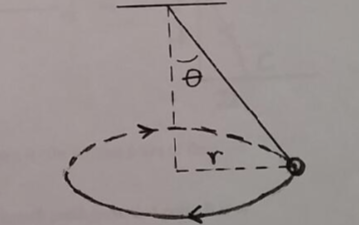# Problem: A ball of mass m = 1.30 kg is attached to a massless rope and swung in a horizontal circle of radius r = 1.70 m, as shown in the figure. The rope makes a constant angle θ with the vertical as the ball swings around the circle, and the ball makes one revolution around the circle in a time Δt = 3.00 s. Make a diagram showing all the forces acting on the ball and the net force acting on the ball. With the help of your diagram, write down equations for the horizontal and vertical forces acting on the ball and use these to determine the angle θ.

###### Problem Details

A ball of mass m = 1.30 kg is attached to a massless rope and swung in a horizontal circle of radius r = 1.70 m, as shown in the figure. The rope makes a constant angle θ with the vertical as the ball swings around the circle, and the ball makes one revolution around the circle in a time Δt = 3.00 s.

Make a diagram showing all the forces acting on the ball and the net force acting on the ball. With the help of your diagram, write down equations for the horizontal and vertical forces acting on the ball and use these to determine the angle θ.